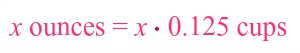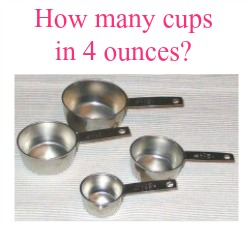# How to Convert Ounces to Cups

Instructor: Laura Pennington

Laura received her Master's degree in Pure Mathematics from Michigan State University, and her Bachelor's degree in Mathematics from Grand Valley State University. She has 20 years of experience teaching collegiate mathematics at various institutions.

Converting from ounces to cups is a relatively easy process. That's what we'll look at in this lesson, as well how to convert the other way round. Finally, we'll see how the conversion process lends itself to real world situations.

## Steps to Solve

Converting from ounces to cups is a relatively easy process. It all falls on the conversion fact that 1 ounce = 0.12503671065 cups. However, I don't know about you, but I think that seems like a lot of digits to work with! Therefore, before, we use this fact to develop the conversion process, let's simplify it a bit by rounding the cups portion to three decimals. That is, 1 ounce = 0.125 cups.

Ah, that's much easier to work with! Okay, let's get to converting! We have that 1 ounce = 0.125 cups. Therefore, if we have x ounces, we can think of that as x copies of 0.125 cups, or x ⋅ 0.125 cups. In a nutshell, this gives us our conversion process. We have that x ounces is equal to x ⋅ 0.125 cups.Therefore, to convert x ounces to cups, we follow these steps;

1. Identify x, or how many ounces we want to convert to cups.
2. Multiply x by 0.125. This converts x ounces to cups.

Told you it was a relatively easy process! It's just a matter of multiplication!

## Solution

To convert x ounces to cups, we use the conversion fact that 1 ounce = 0.125 cups to give that x ounces = x ⋅ 0.125 cups. In other words, to convert x ounces to cups, we simply multiply x by 0.125.

## Application and Going the Other Way

Okay, we know how to convert ounces to cups, which is wonderful! Why, you ask? Well, because this can easily show up in our daily lives. For instance, suppose you are making a casserole that calls for 4 ounces of milk. The problem is that you only have measuring tools of 1 cup, 1/2 cup, 1/3 cup and 1/4 cup, so you don't know how you're going to measure out 4 ounces…or you didn't until reading this lesson! All you have to do is convert 4 ounces to cups, and then you can use the measuring tools you have to measure out the correct amount of milk for your casserole.Okay then, let's do some converting! To convert 4 ounces to cups, all we have to do is multiply 4 ounces by 0.125.

4 ⋅ 0.125 = 0.5

We see that 4 ounces is equal to 0.5 cups, or 1/2 a cup. Great, grab your 1/2 cup measuring tool, and get back to that casserole!

As we said, it's great that we now know how to convert ounces to cups. Here is some more great news! We can use the conversion fact that x ounces = x ⋅ 0.125 cups to figure out how to go the other way, and convert cups to ounces. Watch what happens when we divide both sides of this conversion fact by 0.125.

To unlock this lesson you must be a Study.com Member.

### Register to view this lesson

Are you a student or a teacher?